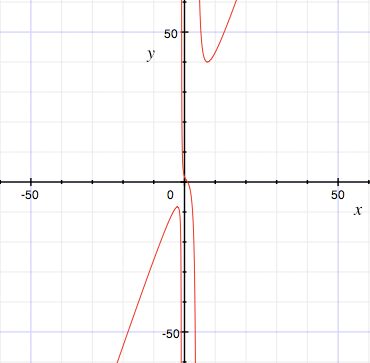SEARCH HOMEMath Central Quandaries & QueriesQuestion from Rachel, a student: How do I find the range of this function? (3x^3-2x^2+6x-7)/(x^2-3x-4)Hi Rachel,

Finding the domain of this function is straightforward. The denominator factors x2 - 3x - 4 = (x - 4)(x + 1) so x = 4 and x = -1 "break" the function. No other values of x cause a problem so the domain is all real numbers x except x = 4 and x = -1.

Determining the range is more of a challenge. As Chris says in the answer to an earlier question

THERE IS NO GENERAL METHOD FOR FINDING THE DOMAIN AND RANGE OF A FUNCTION (except perhaps having a computer draw the graph, and your reading the domain and range from the computer screen!).

I used a computer program to draw a graph.f(x) = (3x3 - 2x2 +6x - 7)/(x2 -3x -4)

From the graph I can see that the range of the function is all real numbers.

I hope this helps,
HarleyMath Central is supported by the University of Regina and The Pacific Institute for the Mathematical Sciences.